# Prepare Cash flow statement in 5 steps

Himanshu Kalra , 01 December 2014

We all have been studying CFS since Class XII, know the theoretical part but when it comes to making Cash flow statements practically, we find ourselves in a jeopardy.

I have been into such situations when the CFS won’t tally, however hard I may try. But with the guidance of my seniors, this problem seems to have been sorted.

So, I have made a 5 step methodology which I am sharing here and believe this could help understanding CFS better.

Step 1

List down values of all assets and liabilities at schedule level for both periods.

Put liability values in negative sign.

So, if you check the total of balance sheet would be zero (Assets – Liabilities)

Now calculate differences between two periods. Ensure that total of differences is also zero.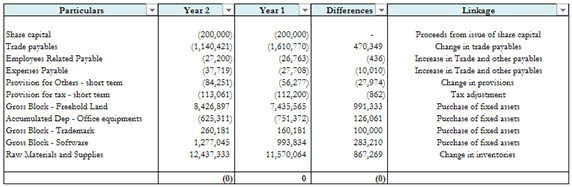Step 2

The next step is to put these differences in the cash flow statement.

For this, write down line item of CFS where the difference should be disclosed.

To discuss few line items:

Difference in assets and liabilities: These would be shown under Net changes in working capital as change in inventory, change in debtors etc.

Difference in share capital: These would be shown as – Proceeds from issue of share capital or repayment of share capital.

Difference in fixed assets: Working needs to be prepared for this to calculate the actual amount of purchase or sale of fixed asset (discussed below).

Difference in retained earnings: The difference would be Profit after tax. This amount adjusted with provision for tax would give PBT.

Difference in borrowings: The difference would be proceeds or repayment from short term borrowings.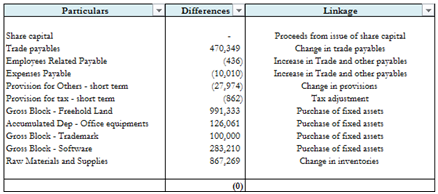Step 3

Now, we need to perform a few calculations w.r.t. some items of our balance sheet to calculate the actual cash inflow/outflow.

a. Fixed asset

Under fixed asset, we would have the opening & closing gross block and opening & closing accumulated depreciation.

So we need to find out actual cash incurred / cash received on purchase or sale of fixed asset respectively.

The fixed asset account as per written down value would be like: (presentation could differ)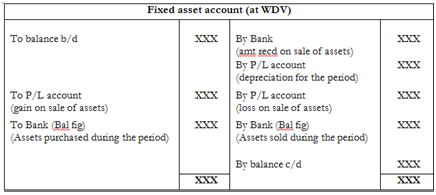The fixed asset account on gross book value would be like: (presentation could differ)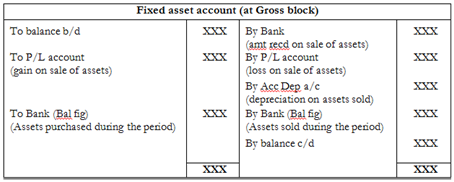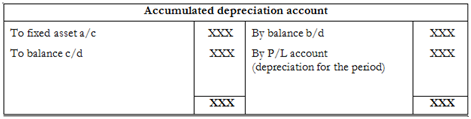b. Interest paid

In the balance sheet we would have the opening and closing interest accrued.

It is not necessary that the interest expense recorded in P/L is also the amount of interest paid during the year. So, we need to find out the actual interest paid (cash outflow) during the period.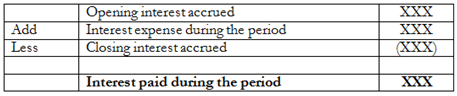In the balance sheet we would have the opening and closing interest earned but not due. Like interest expense, it is not necessary that the interest income recorded in P/L is also the amount of interest received during the year.

So, we need to find out the actual interest received (cash inflow) during the period.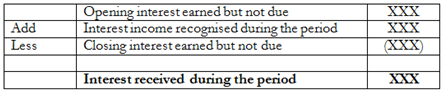d. Taxes paid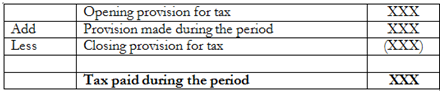Step 4

Now, after all the calculations are done, it’s time to fill the cash flow statement.

Remember, when we identified linkage as to where the difference should go. So, put all the differences one by one in the cash flow statement.

Once all differences are placed, at this point the CFS would tally but it’s not complete, is it?

Now remains the disclosure of non-cash items and few others.

Non-cash items, as the name suggests are those items in which no inflow or outflow of cash takes place.Eg: Depreciation. We treat depreciation as expense in P/L but no cash flow takes place because of it.

Our CFS is tallied at this point, so be careful not to lose control.

Adjust all non-cash items one by one. Let’s take a few examples:

Deprecation:

The fixed asset difference includes asset purchased, asset sold and depreciation for the period.We adjusted depreciation in the fixed asset a/c prepared. So now, add depreciation in the non-cash items.

Interest expense

The interest expense for the period is added in the non-cash adjustments, and interest actually paid is deducted in financing activities.

Interest income

The interest income for the period is deducted in non-cash adjustments and interest received is added in investing activities.

Step 5

Now the last step is to find out whether our calculations tally with the closing cash balance.

Find out the total cash generated from / used in operations by summing up operating, financing and investing activities.

Himanshu Kalra

Himanshu Kalra
(Consultant)
Category Students   Report

9 Likes   151 Shares   74121 Views

### Related Articles### Popular Articles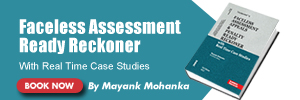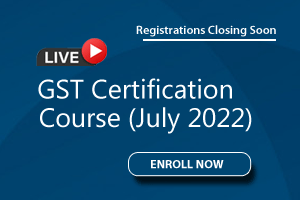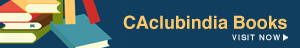CCI Articles